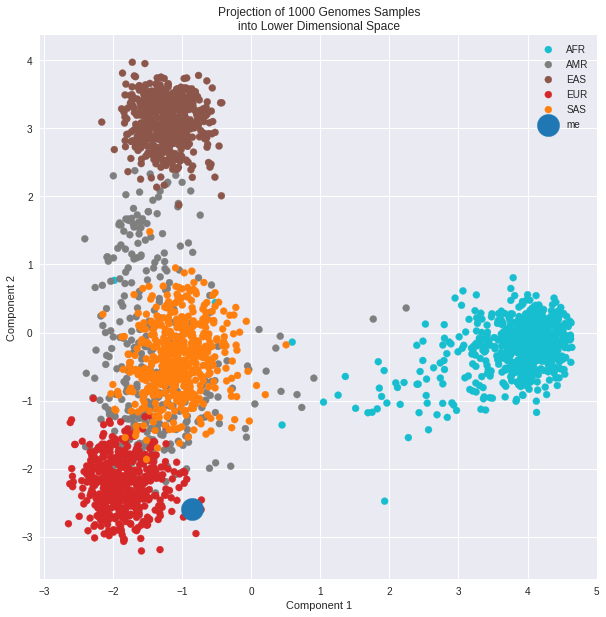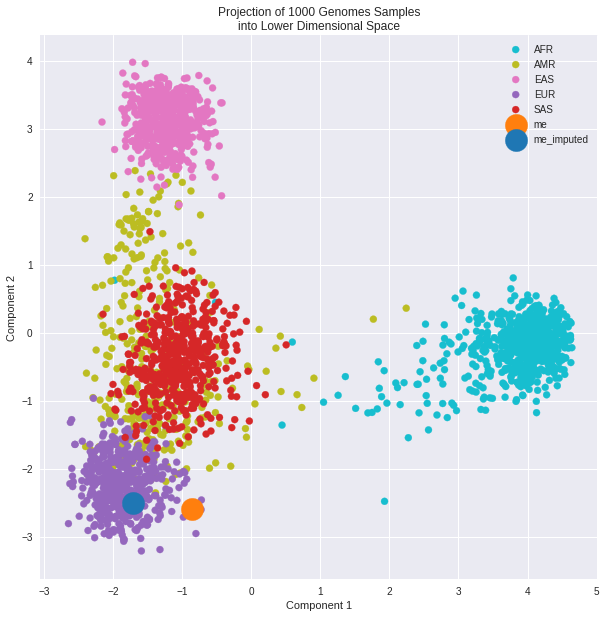# Details for `genome_population_projection.ipynb`

#### Description

Visualize your genotypes in 1000 Genome Project among the population structure.

#### Tags & Data Sources

This notebook really helped me transform yor genotype data into reduced dimensions

# Visualize your genotypes in the 1000 Genomes Project Population Space¶

This notebook will show you how to transform yor genotype data into reduced dimensions using the 1kGP as reference populations.
Dimensionality Redcution techniques include PCA, t-SNE, and UMAP.

If you don't want to click through each cell of this notebook and you only care about the cool visualizations, just select Run All from Cell in the menu bar above.

Also, check out the interactive visualization app.

### Convert a .vcf of the 1000 Genomes Project sample genotypes to a pandas DataFrame¶

The reference `.vcf` has been created and steps documented by subsetting 1kGP sample genotypes to ancestry informative SNPs (AISNPs).

### Choose which AISNPs set to use¶

0 - ref/ref
1 - ref/alt
2 - alt/alt
3 - Unknown

Out:
rs3737576 rs7554936 rs2814778 rs798443 rs1876482 rs1834619 rs3827760 rs260690 rs6754311 rs10497191 ... rs17642714 rs4471745 rs11652805 rs2042762 rs7226659 rs3916235 rs4891825 rs7251928 rs310644 rs2024566
HG00096 0 1 0 2 0 0 0 1 0 2 ... 0 0 1 0 0 2 2 1 1 0
HG00097 0 2 0 2 0 0 0 0 0 2 ... 1 0 2 0 0 2 1 0 0 1
HG00099 0 1 0 1 2 1 0 2 0 2 ... 0 0 1 0 0 1 1 0 0 1

3 rows × 55 columns

Out:
pop super_pop gender
sample
HG00096 GBR EUR male
HG00097 GBR EUR female
HG00099 GBR EUR female

```Found your genotype data in Open Humans!
```

### append your data to the reference data¶

```[pbgzip] member.vcf.gz already exists; do you wish to overwrite (y or n)? [pbgzip] not overwritten
```
Out:
rs3737576 rs7554936 rs2814778 rs798443 rs1876482 rs1834619 rs3827760 rs260690 rs6754311 rs10497191 ... rs17642714 rs4471745 rs11652805 rs2042762 rs7226659 rs3916235 rs4891825 rs7251928 rs310644 rs2024566
NA21143 0 2 0 1 0 0 0 2 1 2 ... 0 0 0 0 0 2 2 0 2 0
NA21144 0 1 0 2 0 2 0 2 2 2 ... 0 0 2 0 1 2 2 0 0 1
me 0 3 0 1 0 0 0 2 3 3 ... 3 0 2 3 0 3 1 1 0 0

3 rows × 55 columns

### Choose the dimensionality reduction algorithm.¶

{PCA, TSNE, or UMAP}

### Choose the population level¶

Superpopulations and populations for the 1kGP are documented here### Compare imputed genotypes to raw 23andMe data¶

```Found your imputed genotype data in Open Humans!
```
```[pbgzip] member.imputed.vcf.gz already exists; do you wish to overwrite (y or n)? [pbgzip] not overwritten
```

# Compare the plot with the imputed genotypes¶Out:
```0    28
3    11
2     8
1     8
Name: me, dtype: int64```
Out:
```0    32
2    12
1    11
Name: me_imputed, dtype: int64```

# Visualize your genotypes in the 1000 Genomes Project Population Space¶

This notebook will show you how to transform yor genotype data into reduced dimensions using the 1kGP as reference populations.
Dimensionality Redcution techniques include PCA, t-SNE, and UMAP.

If you don't want to click through each cell of this notebook and you only care about the cool visualizations, just select Run All from Cell in the menu bar above.

Also, check out the interactive visualization app.

In :
```import bz2
import os
import io
import requests
import pandas as pd
import pysam
import numpy as np
import umap

from io import StringIO
from MulticoreTSNE import MulticoreTSNE as TSNE
from ohapi import api
from sklearn.preprocessing import OneHotEncoder, LabelEncoder
from sklearn.decomposition import PCA
from sklearn.manifold import TSNE
import vcf

import warnings
warnings.filterwarnings('ignore')

# plotting
import matplotlib.pyplot as plt
plt.style.use('ggplot')
import seaborn as sns
%matplotlib inline
```

### Convert a .vcf of the 1000 Genomes Project sample genotypes to a pandas DataFrame¶

The reference `.vcf` has been created and steps documented by subsetting 1kGP sample genotypes to ancestry informative SNPs (AISNPs).

In :
```def vcf2df(vcf_fname):
"""Convert a subsetted vcf file to pandas DataFrame
and return sample-level population data"""
samples = 'ftp://ftp.1000genomes.ebi.ac.uk/vol1/ftp/release/20130502/integrated_call_samples_v3.20130502.ALL.panel'
dfsamples.set_index('sample', inplace=True)
dfsamples.drop(['Unnamed: 4', 'Unnamed: 5'], inplace=True, axis=1)

vcf_file = requests.get(vcf_fname)
buffered = StringIO(vcf_file.content.decode("utf-8"))

df[variant.ID] = [call.gt_type if call.gt_type is not None else 3 for call in variant.samples]

df = df.join(dfsamples, how='outer')
df = df.drop(['pop', 'super_pop', 'gender'], axis=1)

return df, dfsamples
```

### Choose which AISNPs set to use¶

In :
```# Kidd et al.
aisnps = 55

# Kosoy et al.
#aisnps = 128
```
In :
```if aisnps == 55:
vcf_fname = 'https://s3.amazonaws.com/ancestry-snps-1kgp/Kidd.55AISNP.1kG.vcf'
elif aisnps == 128:
vcf_fname = 'https://s3.amazonaws.com/ancestry-snps-1kgp/Seldin.128AISNP.1kG.vcf'

df, dfsamples = vcf2df(vcf_fname)
```

0 - ref/ref
1 - ref/alt
2 - alt/alt
3 - Unknown

In :
```df.head(3)
```
Out:
rs3737576 rs7554936 rs2814778 rs798443 rs1876482 rs1834619 rs3827760 rs260690 rs6754311 rs10497191 ... rs17642714 rs4471745 rs11652805 rs2042762 rs7226659 rs3916235 rs4891825 rs7251928 rs310644 rs2024566
HG00096 0 1 0 2 0 0 0 1 0 2 ... 0 0 1 0 0 2 2 1 1 0
HG00097 0 2 0 2 0 0 0 0 0 2 ... 1 0 2 0 0 2 1 0 0 1
HG00099 0 1 0 1 2 1 0 2 0 2 ... 0 0 1 0 0 1 1 0 0 1

3 rows × 55 columns

In :
```dfsamples.head(3)
```
Out:
pop super_pop gender
sample
HG00096 GBR EUR male
HG00097 GBR EUR female
HG00099 GBR EUR female

In :
```user = api.exchange_oauth2_member(os.environ.get('OH_ACCESS_TOKEN'))

# uncomment the project_source below depending on which you would like to use

project_source = 'direct-sharing-128'
#project_source = 'direct-sharing-129'
#project_source = 'direct-sharing-120'
#project_source = 'direct-sharing-40'
#project_source = 'direct-sharing-131'

# Get a .vcf from OpenHumans
for record in user['data']:
if record['source'] == project_source and '.vcf' in record['basename']:
print('Found your genotype data in Open Humans!')
with open('member.vcf', 'wb') as handle:
try:
try:
try:
textobj = bz2.decompress(datafile.content)
handle.write(textobj)
except OSError:
textobj = gzip.decompress(datafile.content)
handle.write(textobj)
except OSError:
for block in datafile.iter_content(1024):
handle.write(block)
except:
logger.critical('your data source file is malformated')
break
```
```Found your genotype data in Open Humans!
```

### append your data to the reference data¶

In :
```# start with UNKNOWN genotypes == 3 in pyvcf
df.loc['me'] = np.repeat(, df.shape)

dfsamples.loc['me'] = ['me', 'me', 'male']
```
In :
```# read the raw AISNPs tables from the manuscripts, w/ genomic location and rsid
if aisnps == 55:
elif aisnps == 128:
```
In :
```%%bash
pbgzip member.vcf
tabix member.vcf.gz
```
```[pbgzip] member.vcf.gz already exists; do you wish to overwrite (y or n)? [pbgzip] not overwritten
```
In :
```#buffered = StringIO(textobj.decode("utf-8"))

for i, row in dfaim.iterrows():
chrom = row['Chr']
pos = row['Build 37 nt position'].replace(',', '')
rsid = row['dbSNP rs#']
for variant in vcf_reader.fetch(str(chrom), int(pos)-1, int(pos)):
df.loc['me', rsid] = [call.gt_type if call.gt_type is not None else 3 for call in variant.samples]
```
In :
```df.tail(3)
```
Out:
rs3737576 rs7554936 rs2814778 rs798443 rs1876482 rs1834619 rs3827760 rs260690 rs6754311 rs10497191 ... rs17642714 rs4471745 rs11652805 rs2042762 rs7226659 rs3916235 rs4891825 rs7251928 rs310644 rs2024566
NA21143 0 2 0 1 0 0 0 2 1 2 ... 0 0 0 0 0 2 2 0 2 0
NA21144 0 1 0 2 0 2 0 2 2 2 ... 0 0 2 0 1 2 2 0 0 1
me 0 3 0 1 0 0 0 2 3 3 ... 3 0 2 3 0 3 1 1 0 0

3 rows × 55 columns

### One-Hot Encode the DataFrame¶

In :
```ncols = len(df.columns)
ohe = OneHotEncoder(categories=[range(4)] * ncols, sparse=False)

X = ohe.fit_transform(df.values)
```
In :
```def reduce_dim(X, algorithm='PCA', n_components=4):
"""Reduce the dimensionality of the 55 AISNPs
:param X: One-hot encoded 1kG 55 AISNPs.
:type X: array
:param algorithm: The type of dimensionality reduction to perform.
One of {PCA, UMAP, TSNE}
:type algorithm: str
:param n_components: The number of components to return in X_red
:type n_components: int

:returns: The transformed X[m, n] array, reduced to X[m, n_components] by algorithm.
"""

if algorithm == 'PCA':
X_red = PCA(n_components=n_components).fit_transform(X)
elif algorithm == 'TSNE':
# TSNE, Barnes-Hut have dim <= 3
if n_components > 3:
print('The Barnes-Hut method requires the dimensionaility to be <= 3')
return None
else:
X_red = TSNE(n_components=n_components, n_jobs=4).fit_transform(X)
elif algorithm == 'UMAP':
X_red = umap.UMAP(n_components=n_components).fit_transform(X)
else:
return None
return X_red
```

### Choose the dimensionality reduction algorithm.¶

{PCA, TSNE, or UMAP}

In :
```X_emb = reduce_dim(X, algorithm='PCA', n_components=3)
```
In :
```def encode_class(pop_level='pop'):
"""Encode the population lables for plotting."""
le = LabelEncoder()
if pop_level == 'pop':
labels = le.fit_transform(dfsamples['pop'].values)
elif pop_level == 'super_pop':
labels = le.fit_transform(dfsamples['super_pop'].values)
else:
return None
return le, labels
```

### Choose the population level¶

Superpopulations and populations for the 1kGP are documented here

In :
```# pop_level can be either 'pop' or 'super_pop'
le, labels = encode_class(pop_level='super_pop')
```
In :
```def plot_samples(X_emb, x_component=None, y_component=None):
""""""
unique = np.unique(labels)
colors = [plt.cm.tab10_r(i/float(len(unique)-1)) for i in range(len(unique))]
assignments = [colors[i] for i in labels]

plt.figure(figsize=(10,10));
for (i,cla) in enumerate(set(labels)):
s = None
if le.inverse_transform([cla]) == 'me':
s=500
if le.inverse_transform([cla]) == 'me_imputed':
s=500
xc = [p for (j,p) in enumerate(X_emb[:, x_component-1]) if labels[j]==cla]
yc = [p for (j,p) in enumerate(X_emb[:, y_component-1]) if labels[j]==cla]
cols = [c for (j,c) in enumerate(assignments) if labels[j]==cla]
plt.scatter(xc, yc, s=s, c=cols, label=le.inverse_transform([cla]))
plt.legend();
plt.xlabel('Component {}'.format(x_component));
plt.ylabel('Component {}'.format(y_component));
plt.title('Projection of 1000 Genomes Samples\ninto Lower Dimensional Space');
```
In :
```plot_samples(X_emb, x_component=1, y_component=2)
```### Compare imputed genotypes to raw 23andMe data¶

In :
```# using your imputed genotypes from Imputer -- might take a minute to download
for record in user['data']:
if record['basename'] == 'member.imputed.vcf.bz2':
print('Found your imputed genotype data in Open Humans!')
with open('member.imputed.vcf', 'wb') as handle:
datafile = bz2.decompress(datafile)
handle.write(datafile)
break
```
```Found your imputed genotype data in Open Humans!
```
In :
```%%bash
pbgzip member.imputed.vcf
tabix member.imputed.vcf.gz
```
```[pbgzip] member.imputed.vcf.gz already exists; do you wish to overwrite (y or n)? [pbgzip] not overwritten
```

# Compare the plot with the imputed genotypes¶

In :
```me_gts = df.loc['me'].value_counts()
```
In :
```# start with UNKNOWN genotypes == 3 in pyvcf
df.loc['me_imputed'] = np.repeat(, df.shape)

dfsamples.loc['me_imputed'] = ['me_imputed', 'me_imputed', 'male']
```
In :
```myvcf = vcf.Reader(filename='member.imputed.vcf.gz')

for i, row in dfaim.iterrows():
chrom = row['Chr']
pos = row['Build 37 nt position'].replace(',', '')
rsid = row['dbSNP rs#']

for variant in myvcf.fetch(str(chrom), int(pos)-1, int(pos)):
df.loc['me_imputed', rsid] = [call.gt_type if call.gt_type is not None else 3 for call in variant.samples]

ncols = len(df.columns)
ohe = OneHotEncoder(categories=[range(4)] * ncols, sparse=False)

X = ohe.fit_transform(df.values)
X_emb = reduce_dim(X, algorithm='PCA', n_components=3)

le, labels = encode_class(pop_level='super_pop')

plot_samples(X_emb, x_component=1, y_component=2)
```In :
```me_gts_imputed = df.loc['me_imputed'].value_counts()
```
In :
```# The count of 2's is the number of missing genotypes in your un-imputed vcf
me_gts
```
Out:
```0    28
3    11
2     8
1     8
Name: me, dtype: int64```
In :
```# The count of 3's is the number of missing genotypes in your imputed vcf
me_gts_imputed
```
Out:
```0    32
2    12
1    11
Name: me_imputed, dtype: int64```
In [ ]:
```
```